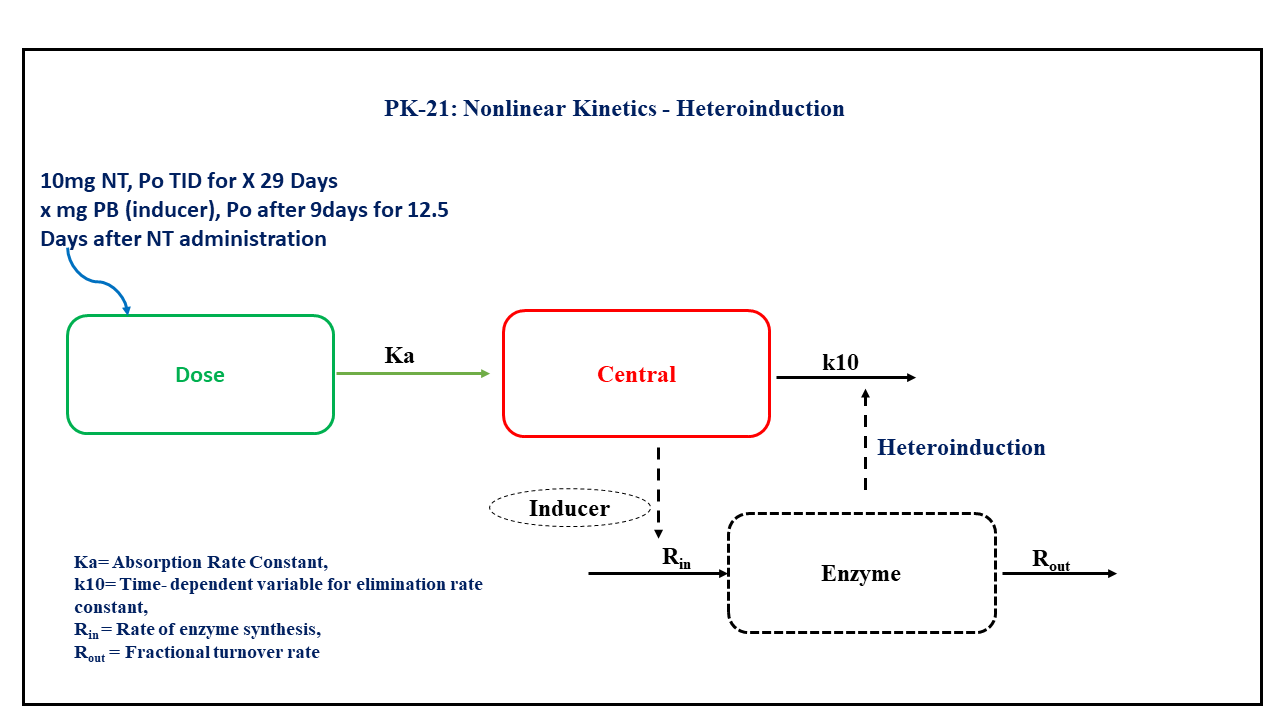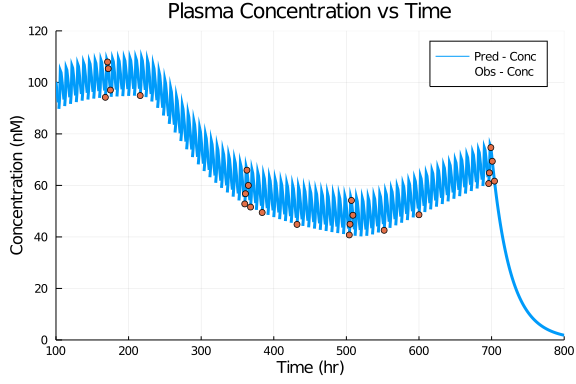# Exercise PK21 - Nonlinear Kinetics - Heteroinduction

### Background

• Structural model - One compartment Oral administration consists of time dependent change in the elimanation rate constant.

• Route of administration - Oral

• Dosage Regimen - Nortriptyline (NT) 10 mg (or 10000 μg) Oral, three times daily for 29 days (i.e. 696 hours), after 216 hours treatment with an enzyme inducer i.e. Pentobarbital (PB) for a period of 300 hours i.e up till 516 hours of treatment with NT.

• Number of Subjects - 1### Learning Outcome

By the application of the present model, we will learn how to simulate model for heteroinduction having first order input/ output model to repeated oral dose data on treatment with an enzyme inducer for a limited duration and how to develop a better fit for the available data.

### Objectives

In this exercise you will learn how to

• Simulate an Oral One Compartment with an enzyme induction consists of time dependent change in the elimination rate constant.

Certain assumptions to be considered:

• The fractional turnover rate i.e. Kout of the enzyme has a longer half-life than the drug or the inducer

• The duration from one level of enzyme activity to other will be influenced by Kout of the enzyme

• The Kout is not regulated by the PB. The interpretation V includes bioavailability (i.e., it is really V/F).

• Write a differential equation for a one-compartment model with oral absorption including time dependent change in elimination rate constant.

### Libraries

call the "necessary" libraries to get start.

using Pumas
using Plots
using CSV
using StatsPlots
using Random


### Model

In this one compartment model, we administer dose in Depot compartment at 'time= 0' that is given every '8 hours' for '87 additional doses'. A second drug which is an enzyme inducer (Pentobarbital) is added at 216 hrs for 300 hrs up to 516 hours of treatment with NT.

Note:- We do not have concentrations of Pentobarbital and hence it is not included in the model.

pk_21        = @model begin
@param begin
tvka     ∈ RealDomain(lower=0)
tvclss   ∈ RealDomain(lower=0)
tvlag    ∈ RealDomain(lower=0)
tvclpre  ∈ RealDomain(lower=0)
tvkout   ∈ RealDomain(lower=0)
tvv      ∈ RealDomain(lower=0)
Ω        ∈ PDiagDomain(3)
σ²_prop  ∈ RealDomain(lower=0)
end

@random begin
η        ~ MvNormal(Ω)
end

@covariates TBP TBP2

@pre begin
Ka       = tvka * exp(η)
Clpre    = tvclpre * exp(η)  # Preinduction Clearance
Clss     = tvclss * exp(η)   # Postinduction Clearance
lags     = (Depot=tvlag,)
Vc       = tvv
Kout     = tvkout
Kpre     = Clpre/Vc
Kss      = Clss/Vc
Kperi    = Kss-(Kss-Kpre)*exp(-Kout*(t-TBP))
A        = Kss - (Kss-Kpre)*exp(-Kout*(TBP2-TBP))
Kpost    = Kpre - (Kpre-A)*exp(-Kout*(t-TBP2))
K10      = (t<TBP) * Kpre + (t>=TBP && t<TBP2) * Kperi + (t>=TBP2) * Kpost
end

@dynamics begin
Depot'   = -Ka*Depot
Central' =  Ka*Depot - K10*Central
end

@derived begin
cp       = @. (1000/263.384)*Central/Vc
dv       ~ @. Normal(cp, sqrt(cp^2*σ²_prop))
end
end

PumasModel
Parameters: tvka, tvclss, tvlag, tvclpre, tvkout, tvv, Ω, σ²_prop
Random effects: η
Covariates: TBP, TBP2
Dynamical variables: Depot, Central
Derived: cp, dv
Observed: cp, dv


### Parameters

The parameters are as given below. tv represents the typical value for parameters.

• Ka - Absorption Rate Constant (1/hr)

• CLss - Intrinsic Clearance post-treatment (L/hr),

• tlag - Lag-time (hrs),

• CLpre - Intrinsic Clearance pre-treatment (L/hr),

• Kout - Fractional turnover rate (1/hr),

• V - Volume of distribution (L),

• Ω - Between Subject Variability,

• σ - Residual error.

param = ( tvka    = 1.8406,
tvclss  = 114.344,
tvlag   = 0.814121,
tvclpre = 46.296,
tvkout  = 0.00547243,
tvv     = 1679.4,
Ω       = Diagonal([0.0,0.0,0.0]),
σ²_prop = 0.015)

(tvka = 1.8406, tvclss = 114.344, tvlag = 0.814121, tvclpre = 46.296, tvkou
t = 0.00547243, tvv = 1679.4, Ω = [0.0 0.0 0.0; 0.0 0.0 0.0; 0.0 0.0 0.0],
σ²_prop = 0.015)


### Dosage Regimen

In this section the Dosage regimen is mentioned for:

• Oral dosing of 10 mg or 10000 μg at time=0 that is given every 8 hours for 87 additional doses for a single subject.

ev1  = DosageRegimen(10000, cmt=1, time=0, ii=8, addl=87)
sub1 = Subject(id=1, events=ev1, covariates=(TBP=216,TBP2=516))

Subject
ID: 1
Events: 88
Covariates: TBP, TBP2


### Simulation

Let's simulate for plasma concentration with the specific observation time points after Oral administration of NT before, during and after treatment with PB.

Random.seed!(123)
sim_sub1 = simobs(pk_21, sub1, param, obstimes=0:1:800)
df1      = DataFrame(sim_sub1)


### Dataframe & Plot

Use the dataframe for plotting

df1_dv = filter(x -> x.time in [0,168,171,172,175,216,360,361,363,365,368,384,432,504,505,507,509,552,600,696,697,699,701,704], df1)

@df df1 plot(:time, :cp,
title= "Plasma Concentration vs Time", label="Pred - Conc",
xlabel="Time (hr)", ylabel="Concentration (nM)",
linewidth=3,  xlims=(100,800), ylims=(0,120),
xticks=[0,100,200,300,400,500,600,700,800], yticks=[0,20,40,60,80,100,120])
@df df1_dv scatter!(:time, :cp, label="Obs - Conc")